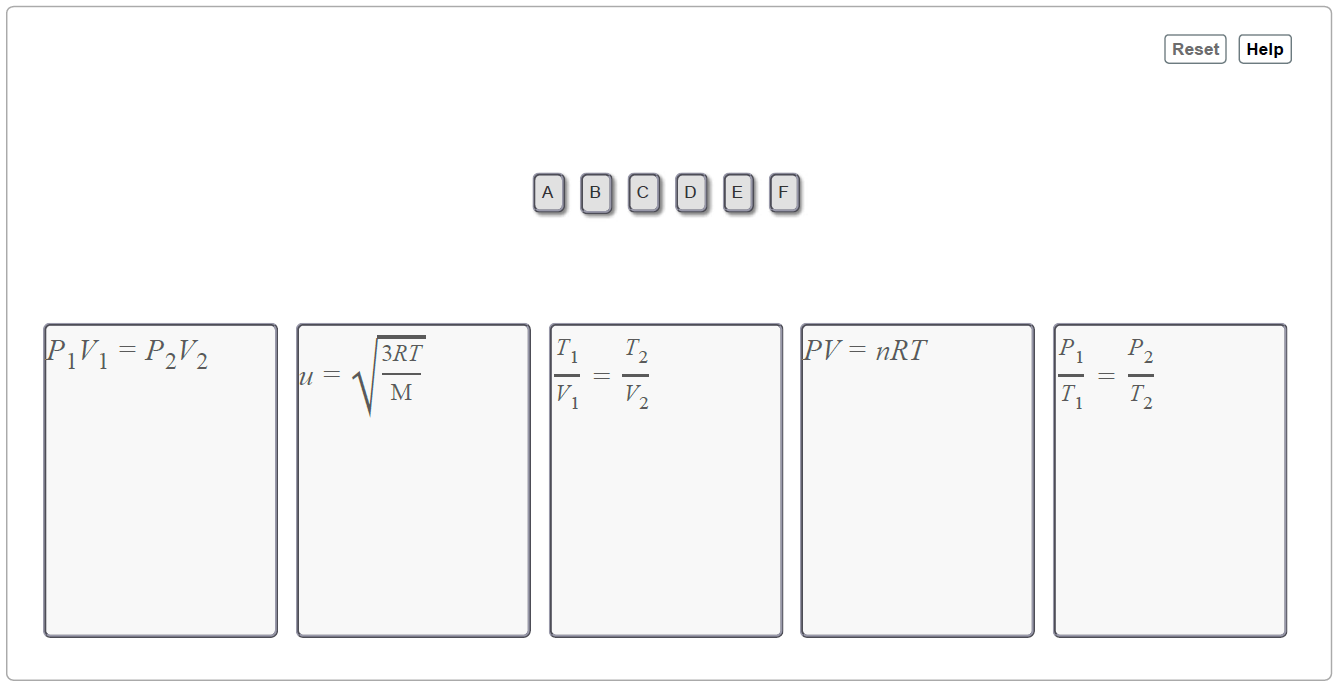# Problem: Classify each situation by the equation that would quantify it.A. A container of ammonia cleaner is stored in the janitor's closet off a lecture hall after being used at night for cleaning. The container has a very small hole in the lid. The next morning the entire lecture hall smells of ammonia. How would you calculate the speed of the ammonia molecules as they move out into the lecture hall?B. The pressure on a tire pump is read. The handle of the pump is pushed down as far as possible. The reading of the pressure on the gauge is taken again. How would you calculate the final pressure?C. The burner under a hot air balloon is ignited and the balloon expands. How would you calculate the expanded volume of the balloon?D. A front-end collision causes the air bag in an automobile to deploy by the reaction of sodium azide to produce nitrogen gas. How would you calculate how much sodium azide is needed to fill a standard size bag with nitrogen under STP conditions?E. Aerosol cans have a label that warns the user not to use them above a certain temperature and not to dispose of them by incineration. Even an empty can contains residual gaseous propellant. If the residual pressure in the can is 1.31 atm when it is sitting on a shelf near a furnace, how could you calculate the pressure when the can is placed on top of the furnace where the temperature reaches the boiling point of water?F. In an automobile engine, at the end of the upstroke the piston is at its maximum height in a cylinder of exact dimensions. At this time, a precisely measured mixture of fuel and oxidant ignites to form gaseous products. If the ignition temperature is known, how could you calculate the pressure inside the cylinder just before the piston moves downward?Drag the appropriate items to their respective bins.A number of empirical relationships or laws exist among the parameters used to describe the behavior of gases (for example, Avogadro's law and the relation of molecular velocity to temperature from kinetic molecular theory). Some of these laws relate initial and final conditions of two changing parameters while all other parameters are held constant (for example, Boyle's law for pressure-volume relationships). Other relationships, such as the ideal gas law, relate parameters without need for initial and final conditions.

###### FREE Expert Solution
87% (398 ratings)View Complete Written Solution
###### Problem Details

Classify each situation by the equation that would quantify it.

A. A container of ammonia cleaner is stored in the janitor's closet off a lecture hall after being used at night for cleaning. The container has a very small hole in the lid. The next morning the entire lecture hall smells of ammonia. How would you calculate the speed of the ammonia molecules as they move out into the lecture hall?
B. The pressure on a tire pump is read. The handle of the pump is pushed down as far as possible. The reading of the pressure on the gauge is taken again. How would you calculate the final pressure?
C. The burner under a hot air balloon is ignited and the balloon expands. How would you calculate the expanded volume of the balloon?
D. A front-end collision causes the air bag in an automobile to deploy by the reaction of sodium azide to produce nitrogen gas. How would you calculate how much sodium azide is needed to fill a standard size bag with nitrogen under STP conditions?
E. Aerosol cans have a label that warns the user not to use them above a certain temperature and not to dispose of them by incineration. Even an empty can contains residual gaseous propellant. If the residual pressure in the can is 1.31 atm when it is sitting on a shelf near a furnace, how could you calculate the pressure when the can is placed on top of the furnace where the temperature reaches the boiling point of water?
F. In an automobile engine, at the end of the upstroke the piston is at its maximum height in a cylinder of exact dimensions. At this time, a precisely measured mixture of fuel and oxidant ignites to form gaseous products. If the ignition temperature is known, how could you calculate the pressure inside the cylinder just before the piston moves downward?

Drag the appropriate items to their respective bins.A number of empirical relationships or laws exist among the parameters used to describe the behavior of gases (for example, Avogadro's law and the relation of molecular velocity to temperature from kinetic molecular theory). Some of these laws relate initial and final conditions of two changing parameters while all other parameters are held constant (for example, Boyle's law for pressure-volume relationships). Other relationships, such as the ideal gas law, relate parameters without need for initial and final conditions.